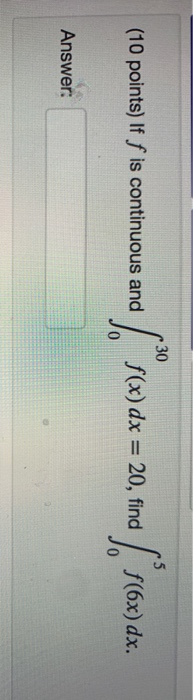# Question Solved1 Answerwith steps please 30 (10 points) If f is continuous and f(x) dx = 20, find JO f(6x) dx. Answer:Transcribed Image Text: 30 (10 points) If f is continuous and f(x) dx = 20, find JO f(6x) dx. Answer:
More
Transcribed Image Text: 30 (10 points) If f is continuous and f(x) dx = 20, find JO f(6x) dx. Answer: